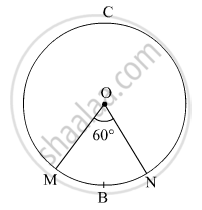Share

# In the Given Figure, Radius of the Circle is 7 Cm and M ( Arc Mbn) = 60°,Find A(O - Mbn) . - Geometry

ConceptPerimeter and Area of a Circle

#### Question

In the given figure, radius of the circle is 7 cm and m ( arc MBN) = 60°,
find  A(O - MBN) .#### Solution

Radius of the circle, r = 7 cm
m(arc MBN) = ∠MON = θ = 60º

Area of the sector = theta/360 xx pir^2

⇒ Area ( O - MBN ) =60/360 × Area of circle

Area of the sector = $\frac{\theta}{360º} \times \pi r^2 = \frac{60º}{360º } \times \frac{22}{7} \times \left( 7 \right)^2$  = 25.7 cm2

A(O- MBN) = 25.7 sq. cm

Is there an error in this question or solution?

#### APPEARS IN

Balbharati Solution for Balbharati Class 10 Mathematics 2 Geometry (2018 to Current)
Chapter 7: Mensuration
Practice set 7.3 | Q: 6.2 | Page no. 154

#### Video TutorialsVIEW ALL 

Solution In the Given Figure, Radius of the Circle is 7 Cm and M ( Arc Mbn) = 60°,Find A(O - Mbn) . Concept: Perimeter and Area of a Circle.
S